Contents >> Applied Mathematics >> Numerical Methods >> Interpolation of Functions >> Newton’s first interpolation formula

 Interpolation of functions - Newton’s first interpolation formula Newton’s first interpolation formula Let in equidistant points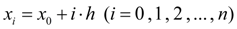, where h – step of interpolation , values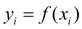are given for function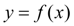. It is required to pick up polynomial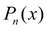of degree not above n , satisfying to conditions (1). Let's enter finite differences for sequence of values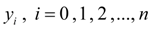: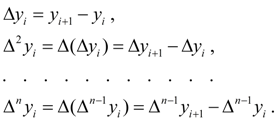(2) Conditions (1) are equivalent to the equalities: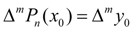at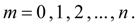Lowering the transformations resulted in [1 ], we’ll finally receive Newton’s first interpolation formula :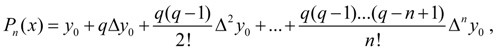(3) where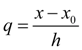– number of steps of interpolation from beginning point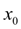up to point х . The formula (3) is expedient for using for interpolation of function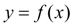in locality of beginning point, where q modulo a little. In special cases we have: at n = 1 – formula of linear interpolation :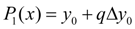; at n = 2 – formula of square-law or parabolic interpolation :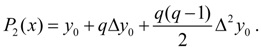< Previous Contents Next >